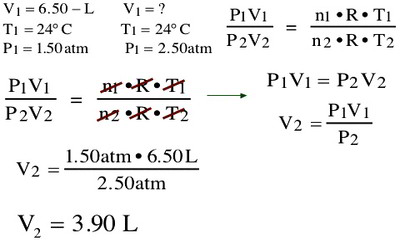Chemistry

# Explain Boyle’s Gas Law

“At constant temperature, the volume of a confined gas is inversely proportional to the pressure to which it is subjected”. (PV = k)

The law itself can be stated as follows:

“For a fixed amount of an ideal gas kept at a fixed temperature, pressure and volume are inversely proportional”

Explanation:

Boyle’s law or the pressure-volume law states that the volume of a given amount of gas held at constant temperature varies inversely with the applied pressure when the temperature and mass are constant.

V is proportional to the inverse of P, PV = C

When pressure goes up, volume goes down. When volume goes up, pressure goes down.  From the equation above, this can be derived:

P1V1 = P2V2 = P3V3 etc.

This equation states that the product of the initial volume and pressure is equal to the product of the volume and pressure after a change in one of them under constant temperature. For example, if the initial volume was 500 mL at a pressure of 760 torr, when the volume is compressed to 450 mL, what is the pressure?
Plug in the values:

P1V1 = P2V2

(760 torr)(500 mL) = P2(450 mL)
760 torr x 500 mL/450 mL = P2 844 torr = P2
The pressure is 844 torr after compression.

Mathematical observationExamples of Boyle’s Gas Law

• Doubling the pressure on a gas halves its volume, as long as the temperature of the gas and the amount of gas aren’t changed.
• The bubbles exhaled by a scuba diver grow as the approach the surface of the ocean. (The pressure exerted by the weight of the water decreases with depth, so the volume of the bubbles increases as they rise.)
• Deep sea fish die when brought to the surface. (The pressure decreases as the fish are brought to the surface, so the volume of gases in their bodies increases, and pops bladders, cells, and membranes).
• Pushing in the plunger of a plugged-up syringe decreases the volume of air trapped under the plunger.